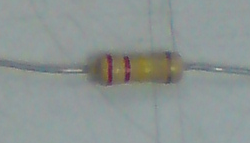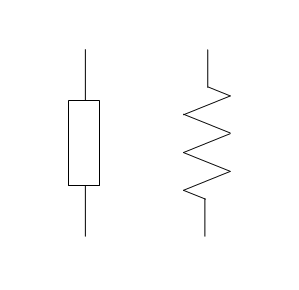## Sample questions resistor color code

1. What is the value of the electrical resistance of the resistor with color rings as follows:
a. Yellow, purple, red, and gold
b. orange, white, brown, and gold
c. green, blue, yellow, and silver
d. brown, black, green, and colorless
e. red, red, red, and gold

2. What color rings on the body of the resistor, the value of resistance mentioned below (ignoring the value of tolerance)?
a. 33 Ω; b. 200 kΩ; c. 750 Ω; d. 43 kΩ; e. 1.2 MΩ

Completion:
1. a. yellow = 4
purple = 7
red = 2
gold = ± 5%
write '4 'and '7', then follow these two numbers with 2 digits '0 '. The results obtained are 4700 Ω or 4.7 kΩ with a tolerance ± 5%

b. orange = 3
white = 9
brown = 1
gold = ± 5%
results obtained is 390 Ω with a tolerance ± 5%

c. Green = 5
blue = 6
yellow = 4
Silver = ± 10%
results obtained are 560,000 Ω or 560 kΩ with a tolerance ± 10%

d. brown = 1
black = 0
Green = 5
colorless = ± 20%
results obtained are 1000000 Ω or 1 MΩ with tolerance ± 20%

e. red = 2
red = 2
red = 2
gold = ± 5%
results obtained is 2200 or 2.2 kΩ with a tolerance ± 5%

2. a. 33 Ω = 33 x 100
3 is orange
3 is orange
0 is black
So ring colors are orange, orange, black.

b. 200 kΩ = 200,000 Ω = 20 x 104
2 is red
0 is black
4 is yellow
So ring the colors are red, black, yellow.

c. 750 Ω = 75 x 101
7 is purple
5 is green
1 is brown
So ring colors are purple, green, brown.

d. 43 kΩ = 43,000 = 43 x 103
4 is yellow
3 is orange
3 is orange
So ring colors are yellow, orange, orange.

e. 1.2 MΩ = 1200 kΩ = 1,200,000 Ω = 12 x 105
1 is brown
2 is red
5 is green
So ring colors are brown, red, green.

## Resistor color code

Four colored rings are used to indicate the resistance value at a fixed resistor. These rings are placed close together at one end of the resistor body. Color of each ring defines a number. See the figure bellowThis color code read from the end nearest resistor, we can know that these colors have meaning:
- The first ring, the first digit of the value of resistance
- The second ring, the second digit of the value of resistance
- The third ring, a multiplier factor (a rank value of 10, or the number of zeros behind the first two digits)
- The fourth ring, is the tolerance of the value of resistance

The table below shows the meaning of the colors on the resistor body

 Color Numbers Black 0 Brown 1 Red 2 Orange 3 Yellow 4 Green 5 Blue 6 Purple 7 Grey 8 White 9 Gold ± 5% Silver ± 10% Without color ± 20%

T7WGAM5GF8TX

## Resistor

In most electrical circuits, we connect the various circuit components using copper wires. This is because copper is an electrical conductor material is excellent. Copper electrical resistance, very low. However, a number of connections on the circuit requires electrical resistance greater than can be provided by copper wire. This is why we need a resistor.

Photo below shows a typical fixed resistor. Type resistors are sold in a range of specific resistance values, ranging from less than 1 Ω to 10 MΩ max.Figure below shows two different symbols, which are used to present the resistors in an electrical circuit diagram.Preference values
Resistors are made with certain values (in units of ohms, or Ω):
1.0 1.1 1.2 1.3 1.5 1.6 1.8 2.0 2.2 2.4 2.7 3.0 3.3 3.6 3.9 4.3 4.7 5.1 5.6 6.2 6.8 7.5 8.2 9.1
After these 24 values, the same sequence of values will be repeated in multiples of tens:
10 11 12 13 ... until ... 91, then
100 110 120 130 ... until ... 910, then
1 k 1.1 k 1.2 ... until ... 9.1 k, then
11 k 10 k 12 k ... until ... 91 k, then
110 k 100 k 120 k ... until ... 910 k, then
1 M 1.1 M 1.2 M ... until ... 9.1 M and 10 M